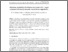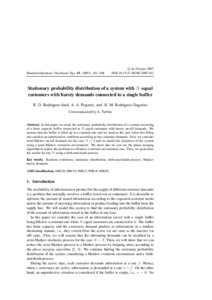# Stationary probability distribution of a system with N equal customers with bursty demands connected to a single buffer

Rodríguez-Saіd, R. D. and Pogoruі, А. А. and Rodríguez-Dagnіno, Ramón М. (2007) Stationary probability distribution of a system with N equal customers with bursty demands connected to a single buffer. Stochastic Eqs (15). pp. 181-204.Preview
Text

## Abstract

In this paper we study the stationary probability distribution of a system consisting of a finite capacity buffer connected to N equal customers with bursty on-off demands. We assume that the buffer is filled up at a constant rate and we analyze the case when this filling rate satisfies an optimization condition according to the customer demands. First, we consider semi-Markov on-off demands for the case N = 2 and we model the dynamics of the system using a semi-Markov evolution environment. We show that we can use the phase merging algorithm to reduce the problem to a Markov evolution environment case. Then, we generalize the results for any N using a birth-and-death process.

Item Type: Article Q Science > QA Mathematics > Mathematical Analysis Faculty of Physics and Mathematics > Department of Mathematical Analysis Ірина Ігорівна Таргонська 21 Oct 2014 07:23 15 Aug 2015 09:11 http://eprints.zu.edu.ua/id/eprint/13247View Item